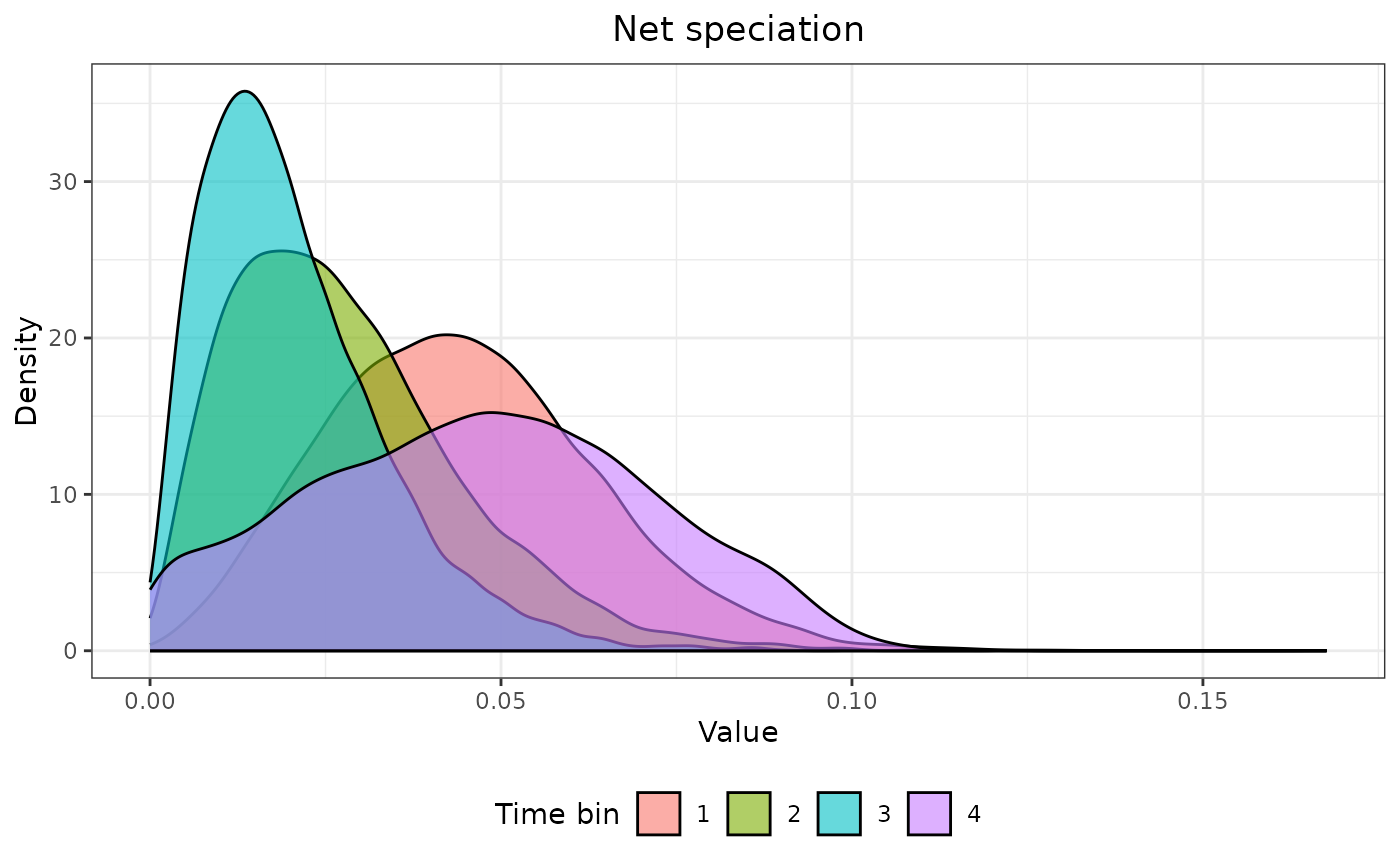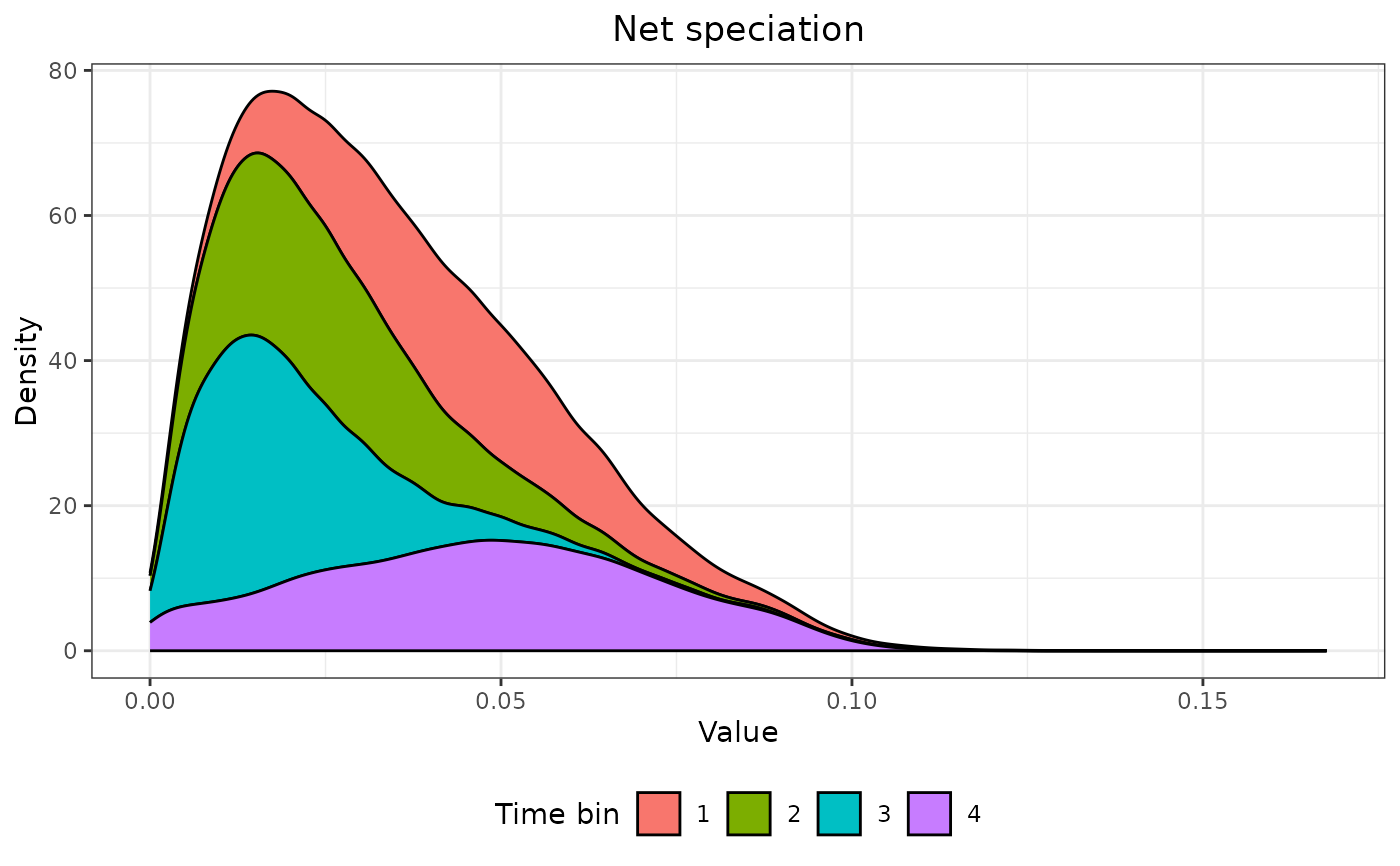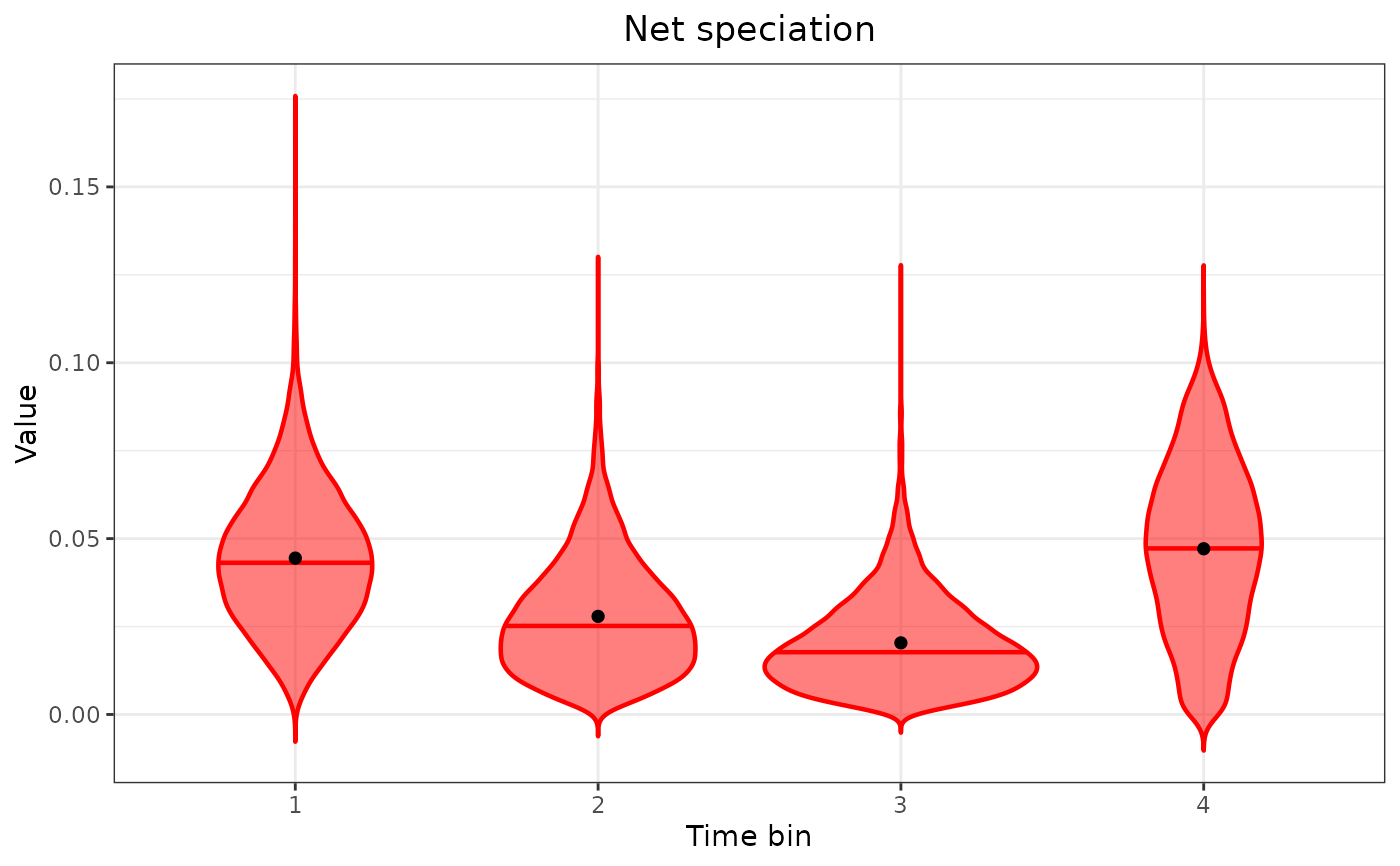Produces a density or violin plot displaying the distribution of FBD parameter samples by time bin.

## Usage

FBD_dens_plot(posterior, parameter, type = "density",
stack = FALSE, color = "red")

## Arguments

posterior

A data frame of posterior parameter estimates containing a single "Time_bin" column and one column for each FBD parameter value. Such data frame can be imported using combine_log followed by FBD_reshape.

parameter

A string containing the name of an FBD parameter in the data frame; abbreviations allowed.

type

The type of plot; either "density" for a density plot or "violin" for violin plots. Abbreviations allowed.

stack

When type = "density", whether to produce stacked densities (TRUE) or overlapping densities (FALSE, the default). Ignored otherwise.

color

When type = "violin", the color of the plotted densities.

## Details

Density plots are produced using ggplot2::stat_density, and violin plots are produced using ggplot2::geom_violin. On violin plots, a horizontal line indicates the median (of the density), and the black dot indicates the mean.

## Value

A ggplot object, which can be modified using ggplot2 functions.

## Note

When setting type = "violin", a warning may appear saying something like "In regularize.values(x, y, ties, missing(ties), na.rm = na.rm) : collapsing to unique 'x' values". This warning can be ignored.

vignette("fbd-params") for the use of this function as part of an analysis pipeline.

ggplot2::stat_density, ggplot2::geom_violin for the underlying functions to produce the plots.

combine_log for producing a single data frame of FBD parameter posterior samples from multiple log files.

FBD_reshape for converting a single data frame of FBD parameter estimates, such as those imported using combine_log, from wide to long format.

FBD_summary, FBD_normality_plot, FBD_tests1, and FBD_tests2 for other functions used to summarize and display the distributions of the parameters.

## Examples

# See vignette("fbd-params") for how to use this
# function as part of an analysis pipeline

data("posterior3p")

posterior3p_long <- FBD_reshape(posterior3p)

FBD_dens_plot(posterior3p_long, parameter = "net_speciation",
type = "density", stack = FALSE)FBD_dens_plot(posterior3p_long, parameter = "net_speciation",
type = "density", stack = TRUE)FBD_dens_plot(posterior3p_long, parameter = "net_speciation",
type = "violin", color = "red")